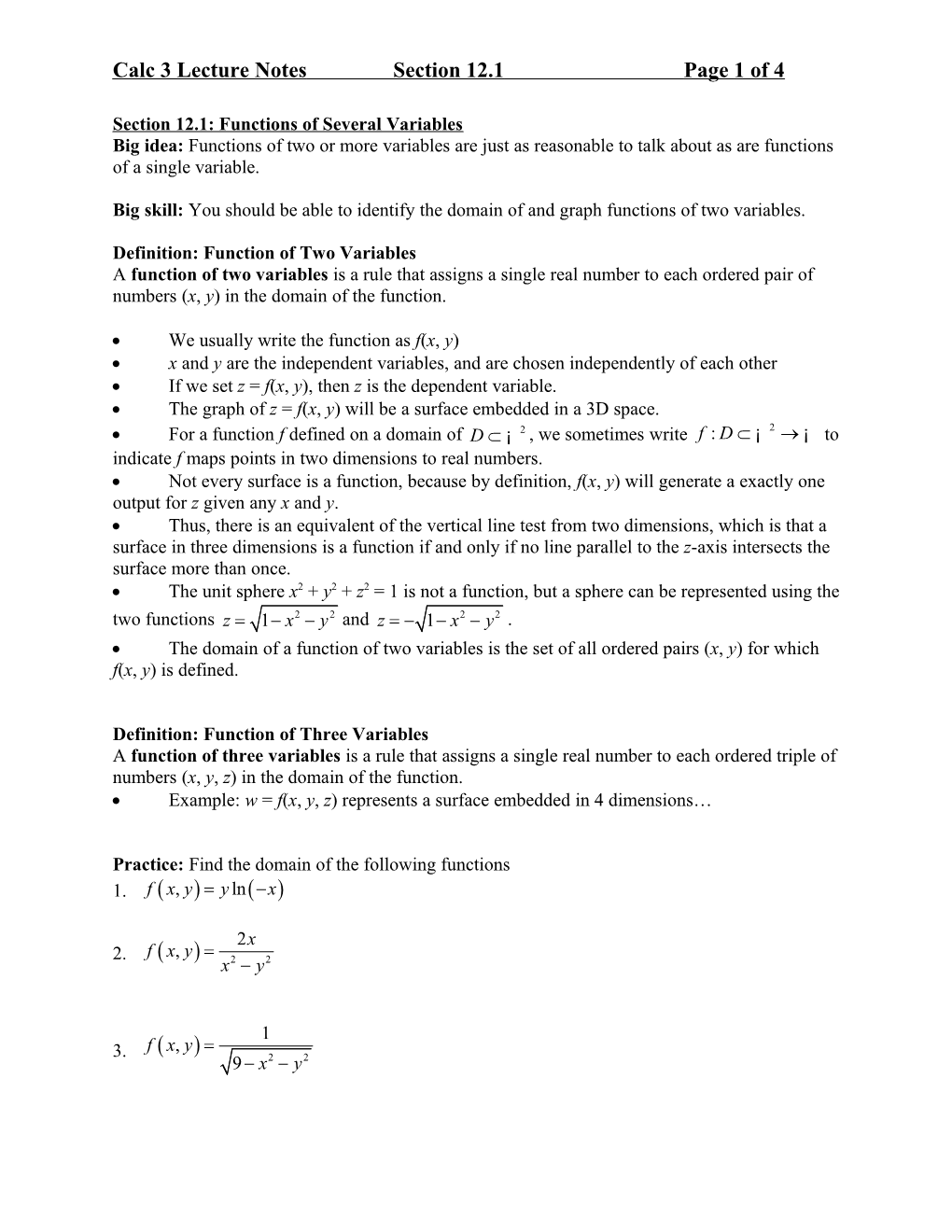# Calculus 3 Lecture Notes, Section 12.1Calc 3 Lecture NotesSection 12.1Page 1 of 4

### Section 12.1: Functions of Several Variables

Big idea: Functions of two or more variables are just as reasonable to talk about as are functions of a single variable.

Big skill: You should be able to identify the domain of and graph functions of two variables.

Definition: Function of Two Variables

A function of two variables is a rule that assigns a single real number to each ordered pair of numbers (x, y) in the domain of the function.

• We usually write the function as f(x, y)
• x and y are the independent variables, and are chosen independently of each other
• If we set z = f(x, y), then z is the dependent variable.
• The graph of z = f(x, y) will be a surface embedded in a 3D space.
• For a function f defined on a domain of , we sometimes write to indicate f maps points in two dimensions to real numbers.
• Not every surface is a function, because by definition, f(x, y) will generate a exactly one output for z given any x and y.
• Thus, there is an equivalent of the vertical line test from two dimensions, which is that a surface in three dimensions is a function if and only if no line parallel to the z-axis intersects the surface more than once.
• The unit sphere x2 + y2 + z2 = 1 is not a function, but a sphere can be represented using the two functions and .
• The domain of a function of two variables is the set of all ordered pairs (x, y) for which f(x, y) is defined.

Definition: Function of Three Variables

A function of three variables is a rule that assigns a single real number to each ordered triple of numbers (x, y, z) in the domain of the function.

• Example: w = f(x, y, z) represents a surface embedded in 4 dimensions…

Practice: Find the domain of the following functions

Winplot for making 3D Graphs:

gGaph of on Winplot using “explicit” mode with :
/ Same graph using “parametric” mode, letting
,
with

Below is a graph of the equation for .Notice how considering traces of the form and helps us to understand the graphContour Plots & Level Curves

Contour plots are 2-dimensional images (drawn in the xy-plane) that provide valuable information about a surface that lives in 3D space. A contour plot consists of a number of level curves for the surface.

Specifically, given a function of two variables, , a level curve is the (2D) graph of the curve , for some constant c. This amounts to intersecting the surface with the place (a plane perpendicular to the z-axis), and projecting the resulting curve of intersection into the xy-plane. If we generate a number of level curves and plot them all on the same set of axes, the resulting graph is called a contour plot.

The figure on the left below is a graph of the paraboloid , plotted on Winplot using “explicit” mode with . On the right is a Winplot-generated contour plot for the surface. The level curves appear to be ellipses, as can easily be confirmed from the equation by setting .

Level curves can be generated on Winplot after graphing the surface by clicking on “levels” in the Inventory window. I told Winplot to generate 10 level curves using equally-spaced constant values of z with a low value of 0 and a high value of 5. After entering the low and high values, click on “auto”, then click on “see all” to view the contour plot.

Much information about the shape of a surface can be gained from the contour plot alone, making contour plots particularly valuable tools, especially if 3D graphing software is not readily available (In this example you could have easily deduced the shapes of the level curves from the equation alone and drawn them by hand).

In this case, for example, without even having a graph of the surface in front of us, we could have deduced from the contour plot that the surface has elliptical traces that get bigger as we increase z. Also, from the fact that successive level curves get closer together as we move away from the origin, we could have deduced that the surface is rising at an ever-faster rate the higher we go.